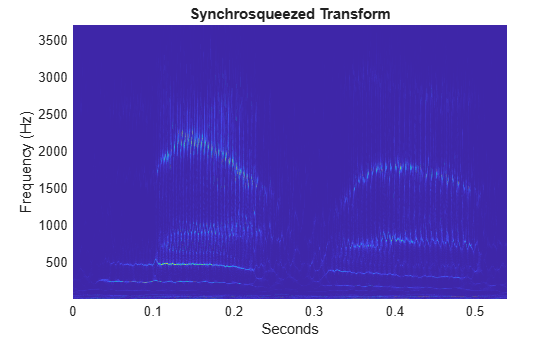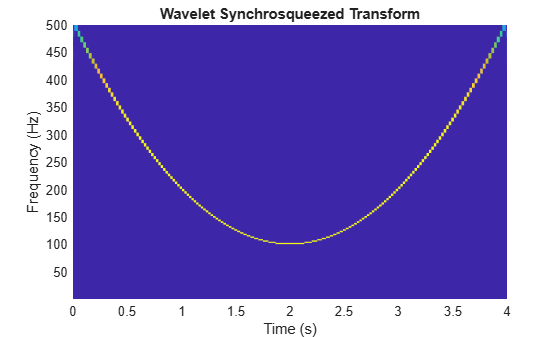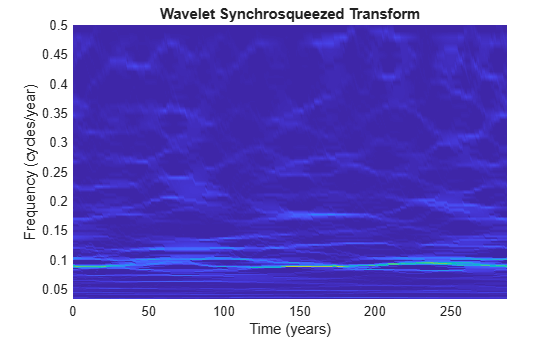# wsst

Wavelet synchrosqueezed transform

## Syntax

``sst = wsst(x)``
``````[sst,f] = wsst(x)``````
``[___] = wsst(x,fs)``
``[___] = wsst(x,ts)``
``[___] = wsst(___,wav)``
``wsst(___)``
``[___] = wsst(___,Name,Value)``

## Description

example

````sst = wsst(x)` returns the wavelet synchrosqueezed transform, `sst`, which you use to examine data in the time-frequency plane. The synchrosqueezed transform has reduced energy smearing when compared to the continuous wavelet transform. The input, `x`, must be a 1-D real-valued signal with at least four samples. `wsst` computes the synchrosqueezed transform using the analytic Morlet wavelet. ```

example

``````[sst,f] = wsst(x)``` returns a vector of frequencies, `f`, in cycles per sample. The frequencies correspond to the rows of `sst`.```

example

````[___] = wsst(x,fs)` computes the synchrosqueezed transform using the specified sampling frequency, `fs`, in Hz, to compute the synchrosqueezed transform. If you specify an `f` output, `wsst` returns the frequencies in Hz. You can use any previous combination of output values.```

example

````[___] = wsst(x,ts)` uses a `duration` `ts` with a positive, scalar input, as the sampling interval. The duration can be in years, days, hours, minutes, or seconds. If you specify `ts` and the `f` output, `wsst` returns the frequencies in `f` in cycles per unit time, where the time unit is derived from specified duration.```

example

````[___] = wsst(___,wav)` uses the analytic wavelet specified by `wav` to compute the synchrosqueezed transform. Valid values are `'amor'` and `'bump'`, which specify the analytic Morlet and bump wavelet, respectively.```
````wsst(___)` with no output arguments plots the synchrosqueezed transform as a function of time and frequency. If you do not specify a sampling frequency, `fs`, or interval, `ts`, the synchrosqueezed transform is plotted in cycles per sample. If you specify a sampling frequency, the synchrosqueezed transform is plotted in Hz. If you specify a sampling interval using a duration, the plot is in cycles per unit time. The time units are derived from the duration.```
````[___] = wsst(___,Name,Value)` returns the synchrosqueezed transform with additional options specified by one or more `Name,Value` pair arguments.```

## Examples

collapse all

Obtain the wavelet synchrosqueezed transform of a speech sample using default values.

```load mtlb; sst = wsst(mtlb);```

Obtain the wavelet synchrosqueezed transform of a speech signal and compare the original and reconstructed signals.

Load the speech signal and obtain its synchrosqueezed transform.

```load mtlb soundsc(mtlb,Fs) dt = 1/Fs; t = 0:dt:numel(mtlb)*dt-dt; [sst,f] = wsst(mtlb,Fs);```

Plot the synchrosqueezed transform.

```pcolor(t,f,abs(sst)) shading interp xlabel('Seconds') ylabel('Frequency (Hz)') title('Synchrosqueezed Transform')```Obtain the inverse synchrosqueezed transform and play the reconstructed speech signal.

```xrec = iwsst(sst); soundsc(xrec,Fs)```

Obtain and plot the wavelet synchrosqueezed transform of a quadratic chirp. The chirp is sampled at 1000 Hz.

```load quadchirp; [sst,f] = wsst(quadchirp,1000); hp = pcolor(tquad,f,abs(sst)); hp.EdgeColor = 'none'; title('Wavelet Synchrosqueezed Transform'); xlabel('Time'); ylabel('Hz');```Obtain the wavelet synchrosqueezed transform of sunspot data using the default Morlet wavelet. Specify the sampling interval to be one year.

```load sunspot wsst(sunspot(:,2),years(1))```Obtain and plot the wavelet synchrosqueezed transform of sunspot data using the bump wavelet. Specify the sampling interval to be 1 for one sample per year.

```load sunspot wsst(sunspot(:,2),years(1),'bump')```## Input Arguments

collapse all

Input signal, specified as a row or column vector. `x` must be a 1-D, real-valued signal with at least four samples.

Sampling frequency, specified as a positive scalar.

Sampling interval, also known as the sampling period, specified as a `duration` with positive scalar input. Valid durations are `years`, `days`, `hours`, `seconds`, and `minutes`. You cannot use calendar durations (`caldays`, `calweeks`, `calmonths`, `calquarters`, or `calyears`). You cannot specify both `ts` and `fs`.

Example: `sst = wsst(x,hours(12))`

Analytic wavelet used to compute the synchrosqueezed transform, specified as one of the following:

• `'amor'` — Analytic Morlet wavelet

• `'bump'` — Bump wavelet

### Name-Value Arguments

Specify optional pairs of arguments as `Name1=Value1,...,NameN=ValueN`, where `Name` is the argument name and `Value` is the corresponding value. Name-value arguments must appear after other arguments, but the order of the pairs does not matter.

Before R2021a, use commas to separate each name and value, and enclose `Name` in quotes.

Example: `'VoicesPerOctave',26`

Number of voices per octave to use in the synchrosqueezed transform, specified as the comma-separated pair consisting of `'VoicesPerOctave'` and an even integer from 10 to 48. The product of the number of voices per octave and the number of octaves is the number of scales. The number of octaves depends on the size of the input `x` and is `floor(log2(numel(x)))-1`.

Option to extend the input signal symmetrically, specified as the comma-separated pair consisting of `'ExtendSignal'` and either `false` or `true`. Extending the signal symmetrically can mitigate boundary effects. If you specify `false`, then the signal is not extended. If you specify `true`, then the signal is extended.

## Output Arguments

collapse all

Synchrosqueezed transform, returned as a matrix. By default, the synchrosqueezed transform uses `floor(log2(numel(x)))-1` octaves, 32 voices per octave, and the analytic Morlet wavelet. `sst` is an Na-by-N matrix where Na is the number of scales, and N is the number of samples in `x`. The default number of scales is `32*(floor(log2(numel(x)))-1)`.

Frequencies of the synchrosqueezed transform, returned as a vector. The frequencies correspond to the rows of the `sst`. If you do not specify `fs` or `ts`, the frequencies are in cycles per sample. If you specify `fs`, the frequencies are in Hz. If you specify `ts`, the frequencies are in cycles per unit time. The length of the frequency vector is the same as the number of `sst` rows. If you specify `ts` as the sampling interval, `ts` is used to compute the scale-to-frequency conversion for `f`.

 Daubechies, I., J. Lu, and H.-T. Wu. "Synchrosqueezed wavelet transforms: an empirical mode decomposition-like tool." Applied and Computational Harmonic Analysis. Vol. 30, Number 2, 2011, pp. 243–261.

 Thakur, G., E. Brevdo, N. S. Fučkar, and H.-T. Wu. "The Synchrosqueezing algorithm for time-varying spectral analysis: robustness properties and new paleoclimate applications." Signal Processing. Vol. 93, Number 5, 2013, pp. 1079–1094.